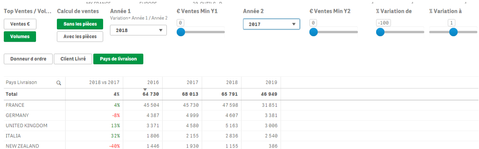# New to Qlik Sense

Discussion board where members can get started with Qlik Sense.

cancel
Showing results for
Did you mean:
HighlightedCreator

## Hide row in straight table - based on calculated field

Hello

I've been looking for days for a solution to get my advanced straight table to work as I wish. The aim of my table is to filter/show only values with a minimum level of sales and a certain variation of sales from one year to another.I have no difficulty to run all calculations based on user selection (I use variables in my calculations).

Nevertheless, I do not manage to hide rows based on conditions.

Conditions could be : 2017 Sales > 2000 and 2018 Sales > 3000 and variation 2018 vs 2017 is between -10% and 0%.

To make it more simple, let's try to focus on showing only rows where sales variation 2018 vs 2017 is between -10% and 0%.

My sales variation is calculated as follow:

=if (([\$(vYearRef1)] > 0 and ([\$(vYearRef2)]=0)),
100,
if (([\$(vYearRef1)] = 0 and ([\$(vYearRef2)]>0)),-1
,([\$(vYearRef1)]/[\$(vYearRef2)])-1))

vYearRef make reference to column names (2018 and 2017 in my example)

I have tried many different possibilities : do not show null values setting all fields to null if conditions are not fullfiled, set limitations (but I can not put a min and max value), aggr functions in the dimension expression...I'm now giving up and asking for your help..

Do you have any idea how to only show countries (rows) where the variation of sales is calculated between -10 and 0%.

Each year sales is calculated thanks to functions:

pick (Vparts,
pick (vAffichage,
sum({<[D_SALES.autoCalendar.#Year]={\$1},[HIERARCHIE]-={"*PIECE*"}>}NET_POSTE),
sum({<[D_SALES.autoCalendar.#Year]={\$1},[HIERARCHIE]-={"*PIECE*"}>}QTE_POSTE)
),

pick (vAffichage,
sum({<[D_SALES.autoCalendar.#Year]={\$1}>}NET_POSTE),
sum({<[D_SALES.autoCalendar.#Year]={\$1}>}QTE_POSTE)

)
)

Each year column calls the above function  (example for 2016):

(\$(vSalesCalculation(\$(=\$(vCurrentYear)-3)

pat

HighlightedCreator

## Re: Hide row in straight table - based on calculated field

Hello

sorry for the head up - I would greatly appreciate if a Qlik expert could let me know if what I intend to do is feasible or not...